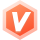2018-10-26 03:22

# 更改主调函数中的变量，使用scanf给变量赋值怎么正确使用？

1,2
x is -92559604281615349000000000000000000000000000000000000000000000.000000,y is -92559604377396321000000000000000000000000000000000000000000000.000000
x value is -92559604281615349000000000000000000000000000000000000000000000.000000,y value is -92559604377396321000000000000000000000000000000000000000000000.000000
min is -92559604377396321000000000000000000000000000000000000000000000.000000,max is -92559604281615349000000000000000000000000000000000000000000000.000000

x value is 1.000000,y value is 2.000000
min is 1.000000,max is 2.000000

#include
#include

void change(double* x, double* y);

int main(void)
{
double a,b;
scanf("%f,%f",&a,&b);

printf("x is %f,y is %f\n",a,b);
change(&a, &b);
a = 1.0;
b = 2.0;
change(&a, &b);

``````system("pause");
``````

}
void change(double* x, double* y)
{
double min,max;
min = *x;
max = *y;
if(*x > *y)
{
min = *y;
max = *x;
}
printf("x value is %f,y value is %f\nmin is %f,max is %f\n",*x,*y,min,max);
}

• 写回答
• 好问题 提建议
• 追加酬金
• 关注问题
• 邀请回答

#### 1条回答默认 最新

•君子黎 2018-10-26 04:31
最佳回答
`````` 1.原因是：
scanf函数是通过指针指向变量的。%f告诉scanf函数在所传地址位置上存储一个float型值，而%lf告诉scanf函数在所传地址位置上存储一个double型值。这里float和double的区别是非常重要的。如果给出了错误的转换说明，那么scanf函数将可能存储错误的字节数量（没有提到的是，float型的为模式可能不同于double型的位模式。
因为double 是64位的，而float是32位的；所以在使用scanf输入double类型时，

``````
`````` 上面的代码需改为：
scanf("%f,%f",&a,&b);---->scanf("%lf,%lf",&a,&b);便可。其他地方都没有问题的。
``````

望采纳！！

评论
解决 无用
打赏 举报